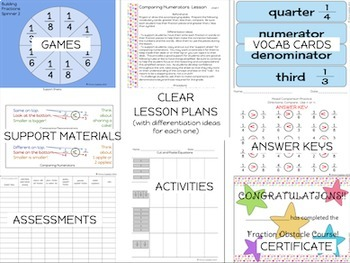3rd, Homeschool
Subjects
Standards
Resource Type
Formats Included
• Zip
Pages
239 pages

### Description

This is a Common Core Standards-based fractions unit. This fraction unit is designed to stand alone and can be taught at any time during the year, and can be combined with any curriculum. It is full of hands-on activities, lessons, and assessments to help your students master ALL of the Common Core fractions standards for 3rd grade. It contains TEN days of fractions lessons, activities, and assessments that will lead your students to mastery of Common Core Fractions Standards 3.NF.1, 2, and 3.
Also appropriate for 4th grade as a review or practice of fractions.

I'm in the process of updating all of my fractions resources for you. I'd love to hear about what you need and want. Share your thoughts on this quick, 2 minute (5 question) survey. Thanks!!

Students will learn:
- fractions concepts (including number line models)
- composing and decomposing fractions
- understanding equivalent fractions
- recognizing equivalent fractions
- finding equivalent fractions on a number line
- generating equivalent fractions
- recognizing fractions that are equivalent to whole numbers
- expressing whole numbers as equivalent fractions
- comparing fractions with the same numerator or denominator
- explaining their reasoning when comparing fractions
- using greater than, less than, or = when comparing fractions
- understanding fractions on a number line
- representing fractions on a number line

Included in this Common Core Fractions unit:
-complete, clear, detailed fraction lesson plans for 10 days
-all necessary fraction printables, worksheets, and slides
-differentiation ideas embedded in EVERY fraction lesson
-fraction explanation writing
-fraction vocabulary cards
-fraction assessment checklist so you can easily track student progress
-fraction end of unit assessment, answer key, and scoring guide
-culminating project: Common Core Fraction Obstacle Course!

Other Common Core and Fraction products you may like:
Common Core Fractions Unit for 4th Grade
Assessments/ Proof of Mastery/ Portfolio Pages for Third Grade
Common Core Geometric Attributes Lesson Plan Unit for 3rd Grade
Common Core Measurement and Line Plot Lesson Plan Unit for 3rd Grade
Common Core Measurement Centers for 3rd Grade
Common Core Summer Packet for 3rd Grade
Holiday Lights Fraction Number Line Activity
FREE Common Core Editable Math Standards Checklists for 3rd Grade
Total Pages
239 pages
Included
Teaching Duration
2 Weeks
Report this Resource to TpT
Reported resources will be reviewed by our team. Report this resource to let us know if this resource violates TpT’s content guidelines.

### Standards

to see state-specific standards (only available in the US).
Compare two fractions with the same numerator or the same denominator by reasoning about their size. Recognize that comparisons are valid only when the two fractions refer to the same whole. Record the results of comparisons with the symbols >, =, or <, and justify the conclusions, e.g., by using a visual fraction model.
Express whole numbers as fractions, and recognize fractions that are equivalent to whole numbers. Examples: Express 3 in the form 3 = 3/1; recognize that 6/1 = 6; locate 4/4 and 1 at the same point of a number line diagram.
Recognize and generate simple equivalent fractions, (e.g., 1/2 = 2/4, 4/6 = 2/3). Explain why the fractions are equivalent, e.g., by using a visual fraction model.
Understand two fractions as equivalent (equal) if they are the same size, or the same point on a number line.
Explain equivalence of fractions in special cases, and compare fractions by reasoning about their size.